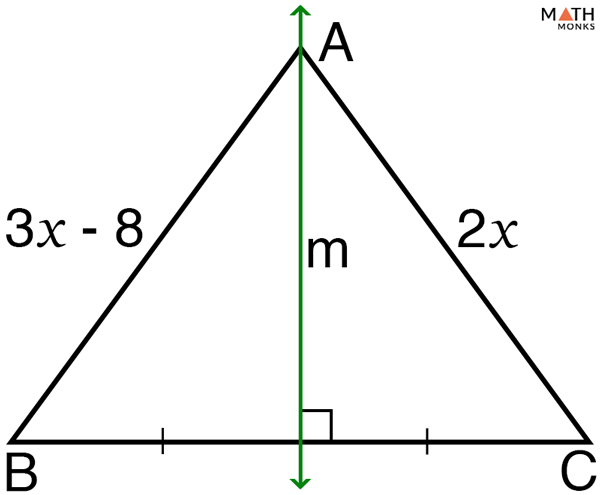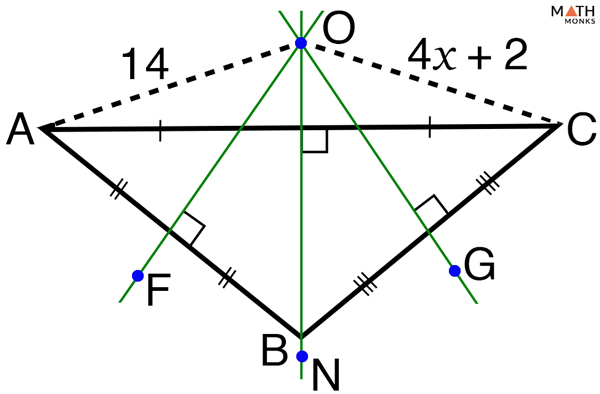# Perpendicular Bisector of a Triangle

Perpendicular bisector is the line segment that intersects another line perpendicularly (at right angle) and divides it into two equal parts. Two lines are perpendicular when they intersect to form 90° with each other, while a bisector divides a line into two equal halves.

## What is a Perpendicular Bisector of a Triangle

The perpendicular bisector of a side of a triangle is a segment, line, or ray perpendicular to that side and dividing the side into two equal parts. In other words, a perpendicular bisector passes through the midpoint of the side it passes through.

There are 3 perpendicular bisectors in a triangle: Ma, Mb, and Mc, each one related to the side it bisects: BC, AC, and AB. The three perpendicular bisectors meet at a point, called the circumcenter. As we know, the point where three or more lines intersect is called the point of concurrency, thus circumcenter is the point of concurrency of the three perpendicular bisectors of a triangle.  The radius (R) of the circumcircle is called the circumradius ‘O’.

The circumcenter is located in different places depending on the type of triangle as follows:

• Found inside the triangle in acute triangles
• Found outside the triangle in obtuse triangles
• Found on the hypotenuse in right triangles

Apart from the above three scenarios, the circumcenter coincides with the midpoint of the hypotenuse in an isosceles right triangle.

## How to Construct a Perpendicular Bisector of a Triangle

To do the perpendicular bisector of a triangle (acute, right or obtuse triangle) we need a ruler and a compass.

Steps

1. Using a ruler, draw a line segment M, whose two ends are AB
2. Open the compass more than half the distance of the line XY and draw two arcs of the same radius centered at A and B
3. The two arcs meet at two points, lets name them P and Q
4. Place the ruler where the two arcs cross and draw the line segment S joining the points P and Q. This is the perpendicular bisector of the side AB

Similarly, draw perpendicular bisectors of all three sides of a triangle: Ma, Mb, and Mc.

## Triangle Perpendicular Bisector Theorem

The Converse of the Triangle Perpendicular Bisector Theorem is also true which states that ‘If the segments created from the vertices to the triangle’s circumcenter are equal, then the circumcenter lies on the intersecting point of the three perpendicular bisectors of the triangle.

## How to Find the Perpendicular Bisector of a Triangle

Let us solve some problems to understand the concept better.Find the value of x. Given m is the perpendicular bisector of BC.

Solution:

If m is the perpendicular bisector of BC, A is equidistant from points B and C
Thus,
=> 3x – 8 = 2x
=> 3x – 2x = 8
=> x = 8Find the value of x in the given triangle.

Solution:

Here, E is the point of concurrency or circumcenter of ΔABC (ΔABC is an obtuse triangle and thus E lies outside the triangle)
As we know,
The circumcenter is equidistant from the vertices.
Then, EA = EC
Thus,
=> 4x +2 = 14
=> 4x = 12
=> x = 3